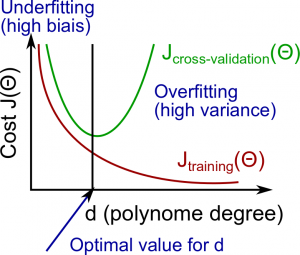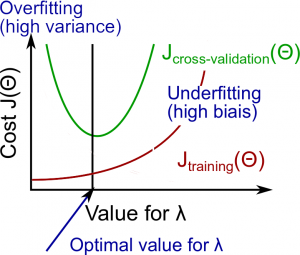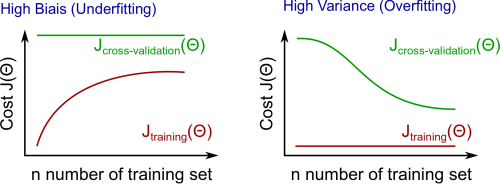β

（转）机器学习笔记7 高偏差/低偏差，学习曲线，模型选择

xge技术博客 408 阅读

Andrew Ng cs229 Machine Learning 笔记

• 获得更多的训练样本
• 减少特征的数量
• 增加特征的数量
• 使用多项式特征
• 增大或减小正则化参数λ

1.评估假设

1. 使用训练集最小化J(Θ)得到Θ参数
2. 计算测试集的误差：
Jtest(Θ)=12mtest∑i=1mtest(hΘ(x(i)test)−y(i)test)2

3.计算分类错误率（即0/1分类错误率）

err(hΘ(x),y)=10if hΘ(x)≥0.5 and y=0 or hΘ(x)<0.5 and y=1otherwise

Test Error=1mtest∑i=1mtesterr(hΘ(x(i)test),y(i)test)

2.模型选择与训练/验证/测试集

• 学习算法若仅仅对训练集拟合较好，并不能说明其假设也是好的。
• 训练集上的假设误差通常要比其他数据集上得到的误差要小。

1. 对不同的多项式次数的模型通过训练集得到最优化参数Θ。
2. 找到在预测集上误差最小的模型的多项式次数d。
3. 使用测试集估计泛化误差Jtest(Θ(d))。

• 训练集：60%
• 交叉验证集：20%
• 测试集：20%

1. 对不同的多项式次数的模型通过训练集得到最优化参数Θ。
2. 找到在验证集上误差最小的模型的多项式次数d。
3. 使用测试集估计泛化误差Jtest(Θ(d))。

3.诊断偏差 Vs. 方差

• 我们需要区分导致预测结果差的原因是偏差还是方差。
• 高偏差也就是欠拟合，高方差也就是过拟合。我们需要在这两者之间找到一个黄金分割。

• 高偏差（欠拟合）：Jtrain(Θ)和JCV(Θ)都较高，并且JCV(Θ)≈Jtrain(Θ)。
• 高方差（过拟合）：Jtrain(Θ)较低，且JCV(Θ)比Jtrain(Θ)高得多。4.正则化和偏差/方差

• λ较大：高偏差（欠拟合）
• λ不大不小：正好
• λ较小：高方差（过拟合）

λ和训练集以及验证集的关系如下：

• λ较小：Jtrain(Θ)较低，且JCV(Θ)较高（高方差/过拟合）。
• λ不大不小：Jtrain(Θ)和JCV(Θ)都较低，并且JCV(Θ)≈Jtrain(Θ)。
• λ较大：Jtrain(Θ)和JCV(Θ)都较高（高偏差/欠拟合）。1. 列出λ测试的值，比如 λ∈{0,0.01,0.02,0.04,0.08,0.16,0.32,0.64,1.28,2.56,5.12,10.24}；
2. 选择一个λ的值进行计算；
3. 创建模型集，比如按照多项式次数或其他指标来创建；
4. 选择一个模型来学习Θ值；
5. 用所选的模型学习得到Θ值，使用选择的λ值计算Jtrain(Θ)（为下一步学习参数Θ）；
6. 使用学习（带λ）得到的参数Θ计算不带正则项或是λ=0的训练误差Jtrain(Θ)；
7. 使用学习（带λ）得到的参数Θ计算不带正则项或是λ=0的交叉验证误差JCV(Θ)；
8. 对模型集合所有λ取值重复上述步骤，选择使交叉验证集误差最小的组合；
9. 如果需要使用图形化结果来帮助决策的话，可以绘制λ和Jtrain(Θ)的图像，以及λ和JCV(Θ)的图像；
10. 使用最好的Θ和λ组合，在测试集上进行预测计算Jtest(Θ)的值来验证模型对问题是否有好的泛化能力。
11. 为了帮助选择最好的多项式次数和λ的值，可以采用学习曲线来诊断。

5.学习曲线

• 当训练集越来越大时，二次函数的误差也会增加。
• 误差值会在训练集大小m增加到一定程度后慢慢平缓。

• 小训练集：Jtrain(Θ)较低，\$J_{CV}(\Theta)较高。
• 大训练集：Jtrain(Θ)和JCV(Θ)都较高，并且J_{train}(\Theta) \approx J_{CV}(\Theta)\$。

• 小训练集：Jtrain(Θ)较低，\$J_{CV}(\Theta)较高。
• 大训练集：Jtrain(Θ)会略微增加，JCV(Θ)会略微降低，并且J_{train}(\Theta) < J_{CV}(\Theta)\$。• 获得更多的训练样本

• 解决高方差
• 减少特征的数量

• 解决高方差
• 增加特征的数量

• 解决高偏差
• 使用多项式特征

• 解决高偏差
• 增加正则参数λ

• 解决高偏差
• 减少正则参数λ

• 解决高方差

7.诊断神经网络

• 参数较少的神经网络很容易欠拟合，但同时计算也较容易。
• 参数较多的大型神经网络更容易过拟合，但同时计算量较大。在这种情况下可以使用正则化（增加λ）来避免过拟合问题。

8.模型选择总结

• 选择多项式次数M
• 如何选择模型中得参数Θ（即模型选择）

1. 获取更多数据（非常困难）
2. 选择拟合数据最好且没有过拟合的模型（非常困难）
3. 通过正则化来减少过拟合的机会

• 高偏差 = 欠拟合（BU）
• Jtrain(Θ)和JCV(Θ)都较高，并且J_{train}(\Theta) \approx J_{CV}(\Theta)\$

• 高方差 = 过拟合（VO）
• Jtrain(Θ)较低，并且Jtrain(Θ)<<JCV(Θ)

• 复杂模型=>数据敏感=>受训练集X变化的影响=>高方差，低偏差
• 简单模型=>更死板=>不受训练集X变化的影响=>低方差，高偏差

• λ值较小（过拟合）使模型容易受噪声影响，导致高方差。
• λ值较大（欠拟合）会将参数值接近于0，导致高偏差。

• 多项式次数较低的模型（模型复杂度低）有高偏差和低方差。在这种情况下，模型拟合总是很差。
• 多项式次数较高的模型（模型复杂度高）拟合训练集极好，拟合测试集极差。导致训练集上低偏差，但高方差。
• 在现实中，我们想要选择一个模型在以上两种情况之间，既然可以很好地拟合数据，也有很好地泛化能力。

• 获取更多地训练样本可以解决高方差问题，不能解决高偏差问题。
• 减少特征数量可以解决高方差问题，不能解决高偏差问题。
• 增加特征数量可以解决高偏差问题，不能解决高方差问题。
• 增加多项式特征和交互特征（特征和特征交互）解决高偏差问题，不能解决高方差问题。
• 当使用梯度下降时，减少正则化参数λ值可以解决高方差问题，增加λ值可以解决高偏差问题。
• 当使用神经网络时，小型神经网络更容易欠拟合，大型神经网络更容易过拟合。交叉验证是选择神经网络大小的一种方式。